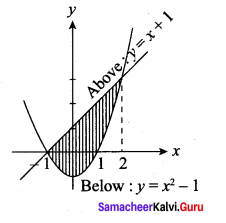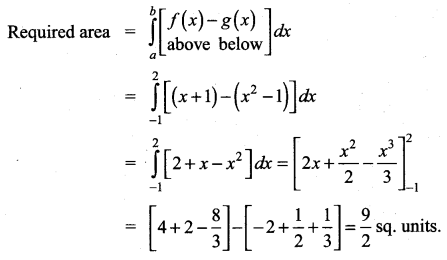## Tamilnadu Samacheer Kalvi 12th Maths Solutions Chapter 9 Applications of Integration Ex 9.8

Question 1.
Find the area of the region bounded by 3x – 2y + 6 = 0, x = -3, x = 1 and x-axis.
Solution: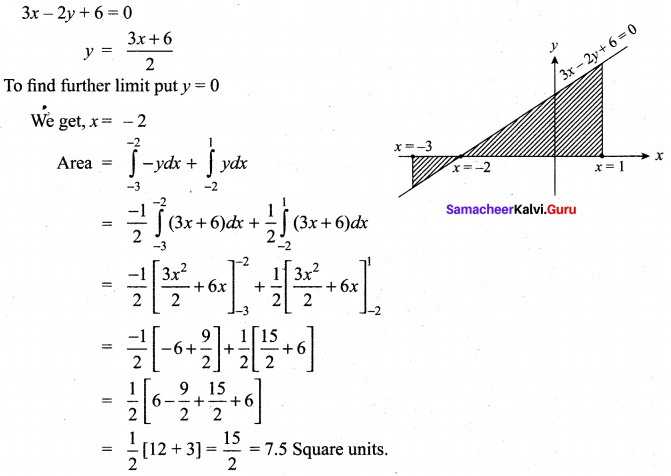Question 2.
Find the area of the region bounded by 2x – y + 1 = 0, y = – 1, y = 3 and y – axis.
Solution:
2x – y + 1 = 0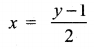To find further limit put x = 0, we get y = 1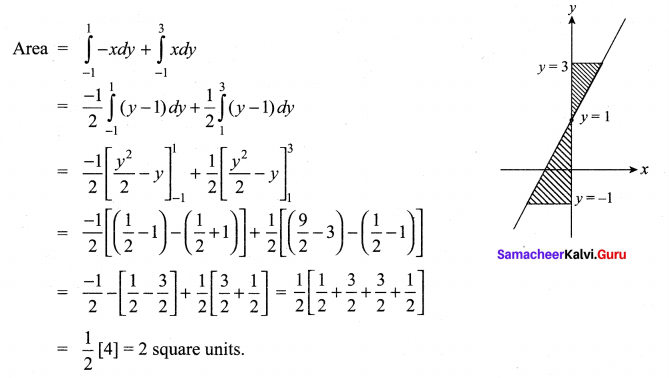Question 3.
Find the area of the region bounded by the curve 2 + x – x2 + y = 0, x – axis, x = – 3 and x = 3.
Solution: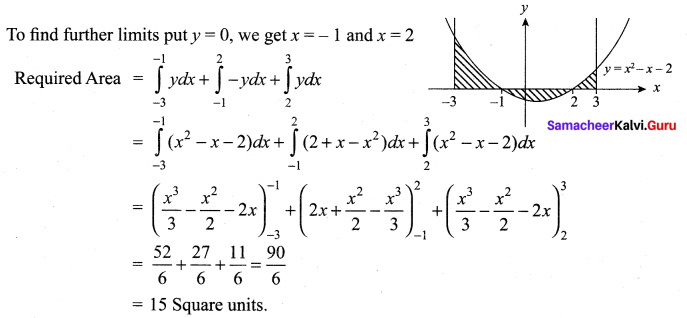Question 4.
Find the area of the region bounded by the line y = 2x + 5 and the parabola y = x2 – 2x.
Solution:
To find point of intersection of the curves
y = 2x + 5 and y = x2 – 2x we get (-1, 3) and (5, 15)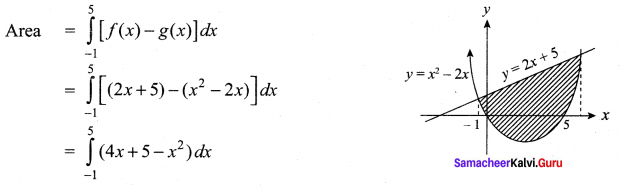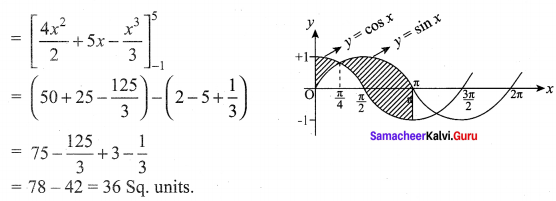Question 5.
Find the area of the region bounded between the curves y = sin x and y = cos x and the lines x = 0 and x = π.
Solution:
To find the points of intersection,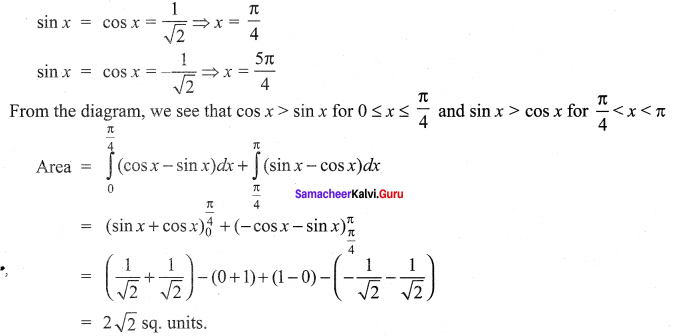Question 6.
Find the area of the region bounded by y = tan x, y = cot x and the line x = 0, x = $$\frac{\pi}{2}$$, 0
Solution:
To find the points of intersection of these two curves between 0 to $$\frac{\pi}{2}$$ is $$\frac{\pi}{4}$$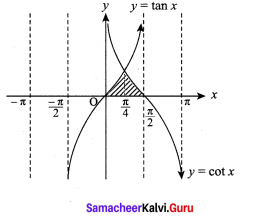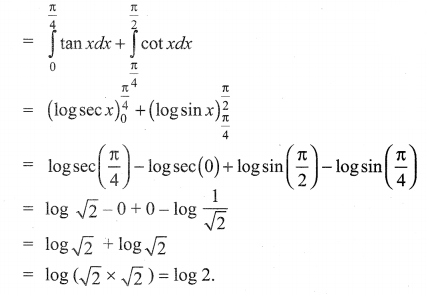Question 7.
Find the area of the region bounded by parabola y2 = x and the line y = x – 2
Solution:
To find the points of intersection solve the two equations y2 = x and y = x – 2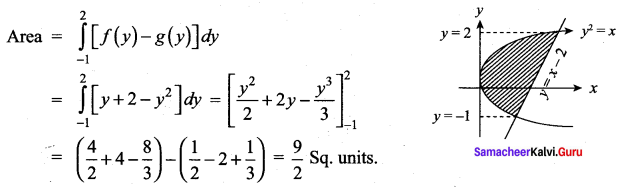Question 8.
Father of a family wishes to divide his square field bounded by x = 0, x = 4 , y = 4 and y = 0 along the curve y2 = 4x and x2 = 4y into three equal parts for his wife, daughter and son. Is it possible to divide? If so, find the area to be divided among them.
Solution:
To find the points of intersection of the two curves, y2 = 4x and x2 = 4y are (0, 0) and (4, 4).
Area of the square field = 4 × 4 = 16 sq. units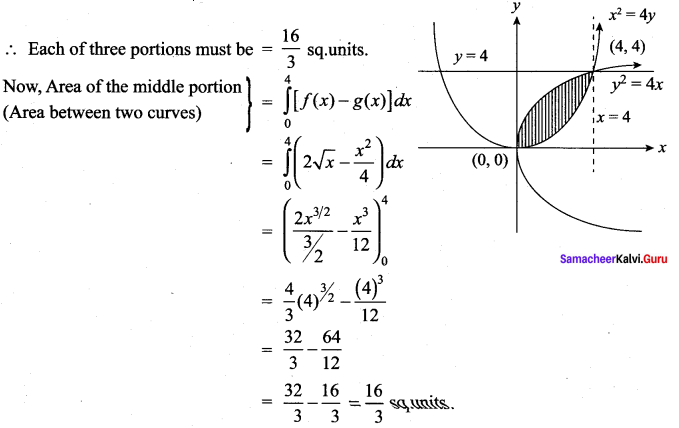So, the remaining each of the two parts must be $$\frac{16}{3}$$ sq.units.
∴ Yes, It is possible to divide the square field into three equal parts.Question 9.
The curves = (x – 2)2 + 1 has a minimum point at P. A point Q on the curve is such that the slope of PQ is 2. Find the area bounded by the curve and the chord PQ.
Solution:
y = (x – 2)2 + 1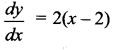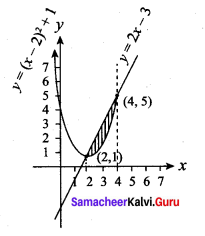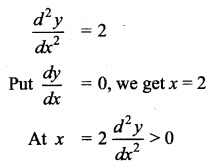∴ x = 2 is a minimum point
∴ The point P is (2, 1)
But slope of PQ is 2
∴ Equation of the chord PQ
y – y1 = m(x – x1)
y – 1 = 2 (x – 2)
y – 1 = 2x – 4
y = 2x – 3
On solving the curve and line we get the point Q(4, 5)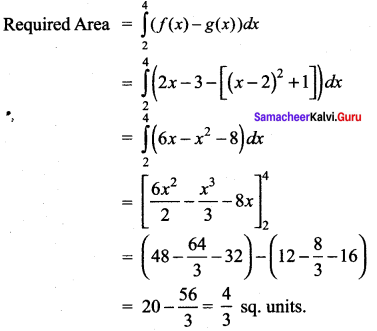Question 10.
Find the area of the region common to the circle x2 + y2 = 16 and the parabola y2 = 6x.
Solution:
To find points of intersection of x2 + y2 = 16 and y2 = 6x are (2, $$2 \sqrt{3})$$) and (2, –$$2 \sqrt{3})$$)
Due to symmetrical property,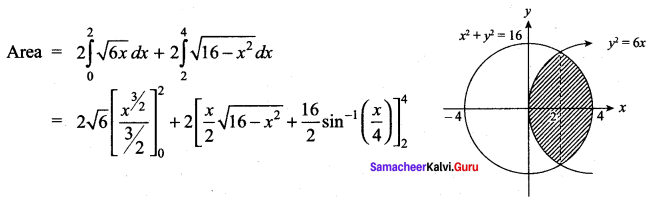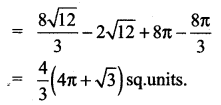### Samacheer Kalvi 12th Maths Solutions Chapter 9 Applications of Integration Ex 9.8 Additional Problems

Question 1.
Find the area of the region enclosed by y2 = x and y = x – 2.
Solution:
The points of intersection of the parabola y2 = x and the line y = x – 2 are (1, -1) and (4, 2)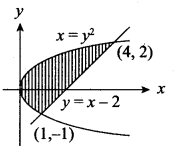To compute the region [shown in figure] by integrating with respect to x, we would have to split the region into two parts, because the equation of the lower boundary changes at x = 1. However if we integrate with respect toy no splitting is necessary.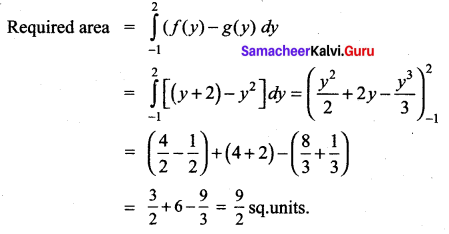Question 2.
Find the area bounded by the curve y = x3 and the line y = x.
Solution:
The line y = x lies above the curve y = x3 in the first quadrant and y = x3 lies above the line y = x in the third quadrant. To get the points of intersection, solve the curves y = x3, y = x ⇒ x3 = x. We get x = {0, ± 1}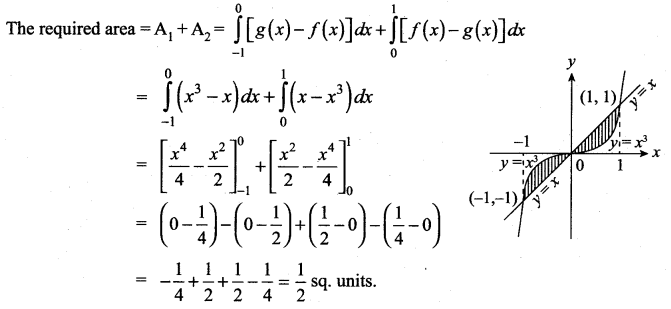Question 3.
Find the area of the loop of the curve 3ay2 = x (x – a)2.
Solution:
Put y = 0; we get x = 0, a
It meets the x – axis at x = 0 and x = a
∴ Here a loop is formed between the points (0, 0) and (a, 0) about x-axis. Since the curve is symmetrical about x-axis, the area of the loop is twice the area of the portion above the x – axis.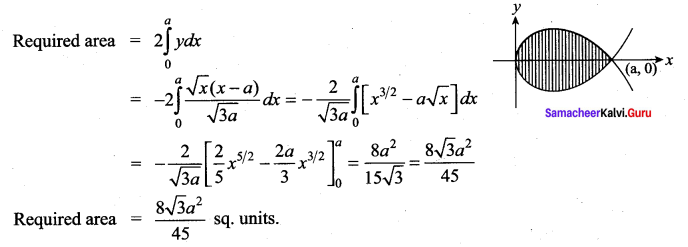Question 4.
Find the area between the line y = x + 1 and the curve y = x2 – 1.
Solution:
To get the points of intersection of the curves we should solve the equations y = x +1 and y = x2 – 1.
we get, x2 – 1 = x + 1
x2 – x – 2 = 0 ⇒ (x – 2)(x + 1) = 0
x = – 1 or x = 2
∴ The line intersects the curve at x = – 1 and x = 2.